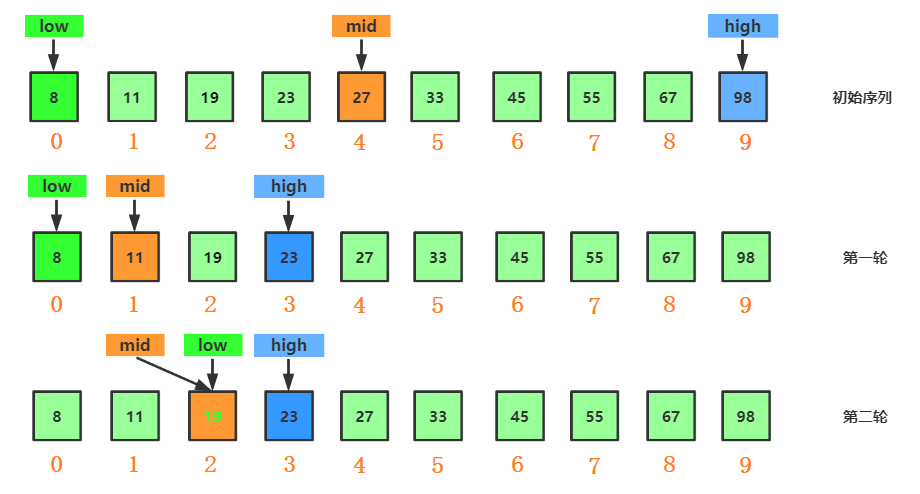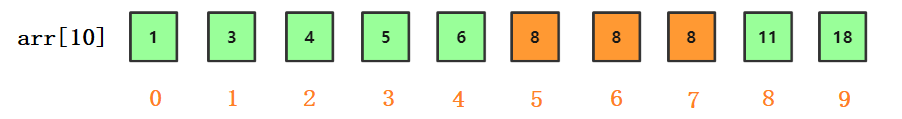二分查找及其变种算法

前言（1）当arr[mid] = value 时，找到则返回 mid下标

（2）当arr[mid] < value 时，说明目标元素在经由mid分割的右区间，故将区间起始位置 low 赋值为mid+1

（3）当arr[mid] > value 时，说明目标元素在经由mid分割的左区间，故将区间结束位置 high 赋值为mid-1复杂度分析编码常规/**
* 迭代法实现
* @param arr
* @param value
* @return
*/
public static int binarySerach1(int[] arr, int  value) {
//头部指针
int low = 0;
//尾部指针
int high = arr.length - 1;

while (low <= high){
int mid = low + ((high - low) >> 1);

if (arr[mid] == value){
return mid;
}else if (arr[mid] > value){
high = mid - 1;
}else {
low = mid + 1;
}
}
return -1;
}

• 循环退出条件：注意是 low <= high，而不是 low < high

• mid 的取值问题：

• mid =( low + high)/2 这种写法是有问题的。因为如果 low 和 high 比较大的话，两者之和就有可能会溢出

• 改进的方法是将 mid 的计算方式写成 low + (high - low)/2

• 将除法运算改为位运算，因为相比除法运算来说，计算机处理位运算要快得多

/**
* 递归法
* @param arr
* @param value
* @return
*/
public static int binarySerach2(int[] arr, int  value){
return bsearch(arr,0,arr.length -1,value);
}

private static int bsearch(int[] arr, int low, int high, int value) {

if (low > high) return -1;

int mid =  low + ((high - low) >> 1);
if (arr[mid] == value) {
return mid;
} else if (arr[mid] > value) {
return bsearch(arr, low, mid-1, value);

} else {
return bsearch(arr, mid+1, high, value);
}
}

变种1、查找第一个值等于给定值的元素public static int binarySerach3(int[] arr, int value) {
int low = 0;
int high = arr.length - 1;
while (low <= high) {
int mid =  low + ((high - low) >> 1);
if (arr[mid] > value) {
high = mid - 1;
} else if (arr[mid] < value) {
low = mid + 1;
} else {
if ((mid == 0) || (arr[mid - 1] != value)) return mid;
else high = mid - 1;
}
}
return -1;
}

• mid == 0，这说明找到的值是数组下标 0 位置的值，也就是起点位置，那么肯定就是第一个值了
• 如果mid前一位的值不是要匹配的value，那么说明找到的就是第一个值，否则，high指向mid - 1，重新比较

2、查找最后一个值等于给定值的元素

/**
* 查找最后一个值等于给定值的元素
* @param arr
* @param value
* @return
*/
public static int binarySerach4(int[] arr, int value) {
int low = 0;
int high = arr.length - 1;
while (low <= high) {
int mid =  low + ((high - low) >> 1);
if (arr[mid] > value) {
high = mid - 1;
} else if (arr[mid] < value) {
low = mid + 1;
} else {
if ((mid == arr.length - 1) || (arr[mid + 1] != value)) return mid;
else high = mid + 1;
}
}
return -1;
}

3、查找第一个大于等于给定值的元素

/**
* 变种三: 查找第一个大于等于给定值的元素
* @param arr
* @param value
* @return
*/
public static int binarySerach3(int[] arr, int value) {
int low = 0;
int high = arr.length - 1;
while (low <= high) {
int mid =  low + ((high - low) >> 1);
if (arr[mid] >= value) {
if ((mid == 0) || (arr[mid - 1] < value)) return mid;
else high = mid - 1;
} else {
low = mid + 1;
}
}
return -1;
}

4、查找最后一个小于等于给定值的元素

/**
* 变种四: 查找第一个大于等于给定值的元素
* @param arr
* @param value
* @return
*/
public static int binarySerach4(int[] arr, int value) {
int low = 0;
int high = arr.length - 1;
while (low <= high) {
int mid =  low + ((high - low) >> 1);
if (arr[mid] > value) {
high = mid - 1;
} else {
if ((mid == arr.length - 1) || (arr[mid + 1] > value)) return mid;
else low = mid + 1;
}
}
return -1;
}

局限性1、二分查找依赖的是顺序表结构，简单点说就是数组

2、二分查找针对的是有序数据

3、数据量太小不适合二分查找。

4、数据量太大也不适合二分查找。

声明